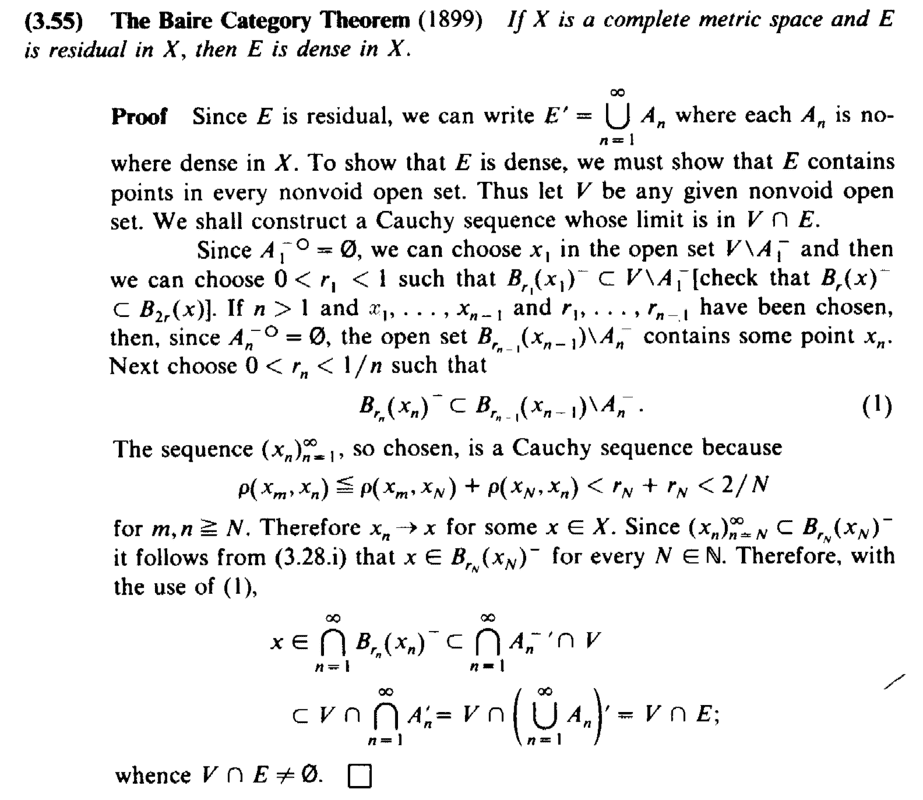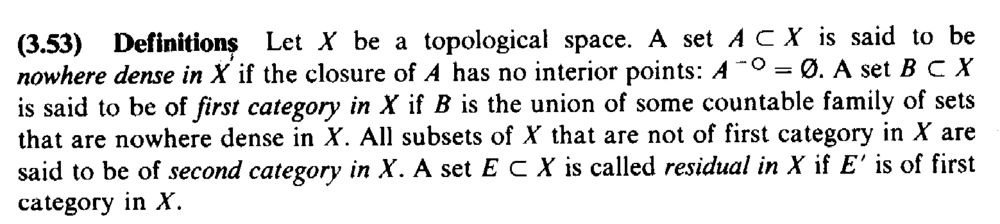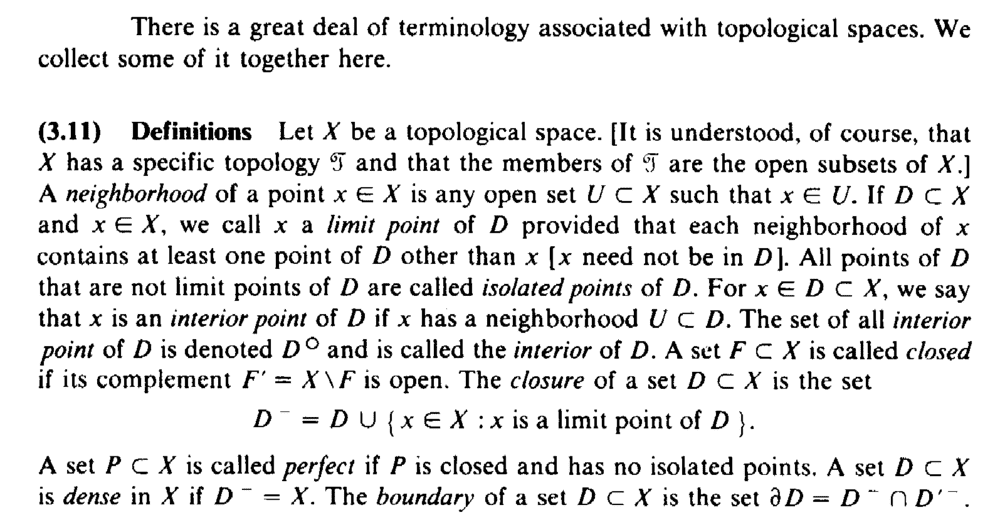# Baire Category Theorem .... Stromberg, Theorem 3.55 .... ....

Gold Member
MHB
TL;DR Summary
The post concerns an aspect of Stromberg's proof of the Baire Category Theorem ... ...
I am reading Karl R. Stromberg's book: "An Introduction to Classical Real Analysis". ... ...

I am focused on Chapter 3: Limits and Continuity ... ...

I need help in order to fully understand the proof of Theorem 3.55 on page 110 ... ...

Theorem 3.55 and its proof read as follows:At the start of the second paragraph of the above proof by Stromberg we read the following:

" ... ...Since ##A_1^{ - \ \circ } = \emptyset##, we can choose ##x_1## in the open set ##V## \ ##A_1^{ - }## and then we can choose ##0 \lt r_1 \lt 1## such that ##B_{ r_1 } ( x_1 )^{ - } \subset V## \ ##A_1^{ - }## [ check that ##B_r (x)^{ - } \subset B_{ 2r } (x) ## ] ... ...

My questions are as follows:

Question 1

Can someone explain and demonstrate exactly/rigorously why/how it is that ##A_1^{ - \ \circ } = \emptyset## means that we can choose ##x_1## in the open set ##V## \ ##A_1^{ - }## ... it seems very plausibly the case ... but ... how are we (rigorously) sure this is true ... ?

Question 2

How/why can we choose ##0 \lt r_1 \lt 1## such that ##B_{ r_1 } ( x_1 )^{ - } \subset V## \ ##A_1^{ - }## ... ?

... and why are we checking that ##B_r (x)^{ - } \subset B_{ 2r } (x)## ... ... ?

*** EDIT ***

My thoughts on Question 2 ...

Since ##V## \ ##A_1^{ - }## is open ... ##\exists \ r_1## such that ##B_{ r_1 } ( x_1 ) \subset V## \ ##A_1^{ - }## ...

... BUT ... how do we formally and rigorously show that ...

... we can choose an ##r_1## such that the closure of ##B_{ r_1 } ( x_1 )## is a subset of ##V## \ ##A_1^{ - }## ... ( ... intuitively we just choose ##r_1## somewhat smaller yet ... )

... and further why is Stromberg talking about ##r_1## between ##0## and ##1## ...?

Help will be much appreciated ...

Peter

==========================================================================================================================

The definitions of nowhere dense, first and second category and residual are relevant ... so I am providing Stromberg's definitions ... as follows:Stromberg's terminology and notation associated with the basic notions of topological spaces are relevant to the above post ... so I am providing the text of the same ... as follows:Hope that helps ...

Peter

Last edited:

Homework Helper
Gold Member
On the first one:

Assume the opposite: that V\A1- is empty. Then A1- contains V, which is a nonempty open set and has interior points (since all points in an open set are interior). So A1- has interior points, but it is the closure of A1, and that contradicts the statement that A1 is nowhere dense. From this contradiction we conclude that V\A1- cannot be empty.

•Math Amateur
Homework Helper
Gold Member
On the second one, note that V\A1- is open because it is the intersection of open set V with the complement of A1-, which is open because A1- is closed. Since it is open, all its points are interior and in particular x1 is, so there exists some h such that the ball Bh(x1) is contained in V\A1- .

He requires r1<1 because a little further down he requires that rn < 1/n. That's how he makes the sequence Cauchy.

•Math Amateur
Gold Member
MHB
Thanks so much for the help, Andrew

Just working through your posts now ...

Thanks again,

Peter

Gold Member
MHB
On the second one, note that V\A1- is open because it is the intersection of open set V with the complement of A1-, which is open because A1- is closed. Since it is open, all its points are interior and in particular x1 is, so there exists some h such that the ball Bh(x1) is contained in V\A1- .

He requires r1<1 because a little further down he requires that rn < 1/n. That's how he makes the sequence Cauchy.

Thanks again for the help Andrew ...

... but ... just a clarification ...

... how exactly do we demonstrate rigorously .. given the ball ##B_h (x_1) \subset V## \ ##A_1^{ - }##

... we then have ##B_h (x_1)^{ - } \subset V## \ ##A_1^{ - }## ... ... ?

Hoe you can help further ...

Peter

•